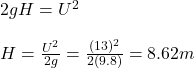A volley ball is hit directly toward the ceiling in a gymnasium with a ceiling height of L 0 m. If the initial vertical velocity is 13 m/s a

Question

A volley ball is hit directly toward the ceiling in a gymnasium with a ceiling height of L 0 m. If the initial vertical velocity is 13 m/s and the release height is 1.8 m will the ball hit the ceiling?

in progress 0
5 months 2021-09-02T05:04:17+00:00 1 Answers 0 views 0

1. Complete Question:

A volley ball is hit directly toward the ceiling in a gymnasium with a ceiling height of 10 m. If the initial vertical velocity is 13 m/s and the release height is 1.8 m will the ball hit the ceiling?

The ball will hit the ceiling

Explanation:

Given;

Initial vertical Velocity U = 13 m/s

Height of the ceiling = 10 m

Released height of the volley ball = 1.8 m

Height traveled by the volley ball, is calculated as follows;where;

V is final vertical velocityRemember this ball was released from 1.8 m height and it traveled 8.62 m.

Total distance traveled = 1.8 + 8.62 = 10.42 m

Therefore, the ball will hit the ceiling